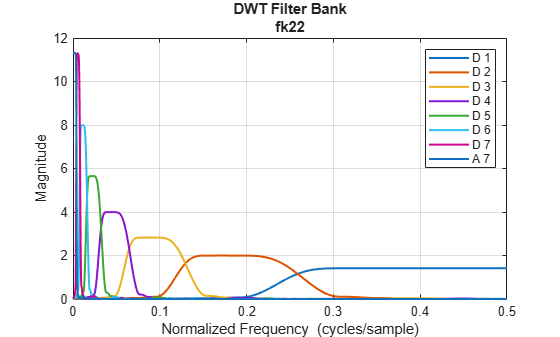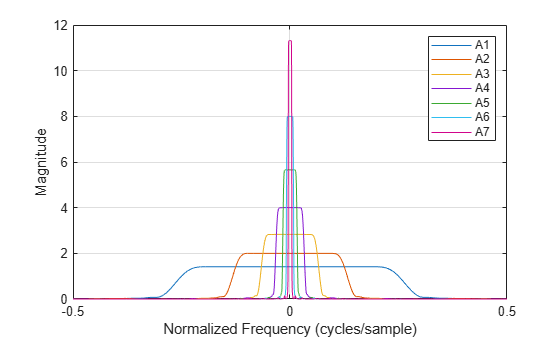Main Content

# freqz

DWT filter bank frequency responses

## Syntax

``[psidft,f] = freqz(fb)``
``[psidft,f,phidft] = freqz(fb)``
``freqz(fb)``

## Description

example

````[psidft,f] = freqz(fb)` returns the complex-valued frequency responses for the wavelet filters `psidft` and the frequency vector `f` for the discrete wavelet transform (DWT) filter bank `fb`. Frequencies are in cycles/sample or in Hz if a sampling frequency is defined in `fb`. The frequency responses are centered so that the zero frequency is in the middle.```
````[psidft,f,phidft] = freqz(fb)` returns the complex-valued frequency responses for the scaling filters `phidft` for the DWT filter bank `fb` at all levels of the decomposition.```
````freqz(fb)` plots the one-sided magnitude frequency responses for the wavelet filter bank, `fb`. Magnitude frequency responses are plotted for all wavelet bandpass filters and the coarsest resolution scaling filter. The legend is interactive. To toggle the visibility of the filter magnitude response, click the corresponding line in the legend.```

## Examples

collapse all

Create a DWT filter bank for a length 4096 signal and the Fejér-Korovkin `fk22` wavelet. Plot the magnitude frequency responses of the wavelet filters and final resolution scaling filter.

```len = 4096; fb = dwtfilterbank('Wavelet','fk22','SignalLength',len); freqz(fb)```

Obtain the frequency responses for the wavelet and scaling filters. Plot the magnitude frequency responses of the scaling filters at all levels of decomposition.

```[psidft,f,phidft] = freqz(fb); plot(f,abs(phidft)') grid on xlabel('Normalized Frequency (cycles/sample)') ylabel('Magnitude') legend('A1','A2','A3','A4','A5','A6','A7')```Plot the one-sided magnitude frequency responses of the wavelet and scaling filters at the first two levels of decomposition. Note how the second level frequency responses overlap the magnitude response of the first level scaling filter.

```plot(f(len/2:end),abs(psidft(1,len/2:end))') hold on plot(f(len/2:end),abs(phidft(1,len/2:end))') plot(f(len/2:end),abs(psidft(2,len/2:end))') plot(f(len/2:end),abs(phidft(2,len/2:end))') grid on xlabel('Normalized Frequency (cycles/sample)') ylabel('Magnitude') legend('Level 1 Wavelet','Level 1 Scaling','Level 2 Wavelet','Level 2 Scaling')```## Input Arguments

collapse all

Discrete wavelet transform (DWT) filter bank, specified as a `dwtfilterbank` object.

## Output Arguments

collapse all

Wavelet filter frequency responses for the DWT filter bank `fb`, returned as an L-by-N matrix, where L is the filter bank `Level` and N is the filter bank `SignalLength`. The frequency responses are centered so that the zero frequency is centered in the middle.

Frequencies, in cycles/sample or Hz, returned as a real-valued vector of length N, where N is the filter bank `SignalLength`. If a sampling frequency is specified in `fb`, frequencies are in Hz.

Data Types: `double`

Scaling function frequency responses for the DWT filter bank `fb`, returned as an L-by-N matrix, where L is the filter bank `Level` and N is the filter bank `SignalLength`. The frequency responses are centered so that the zero frequency is centered in the middle.

## See Also

Introduced in R2018a

## SupportGet trial now